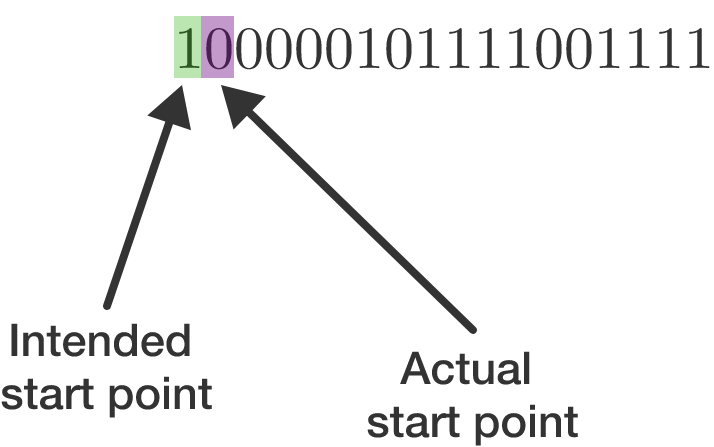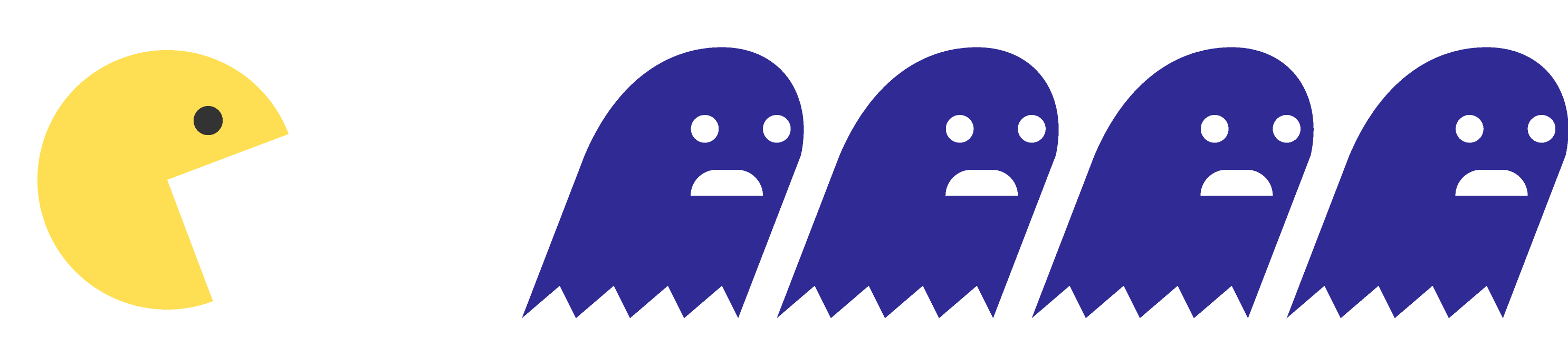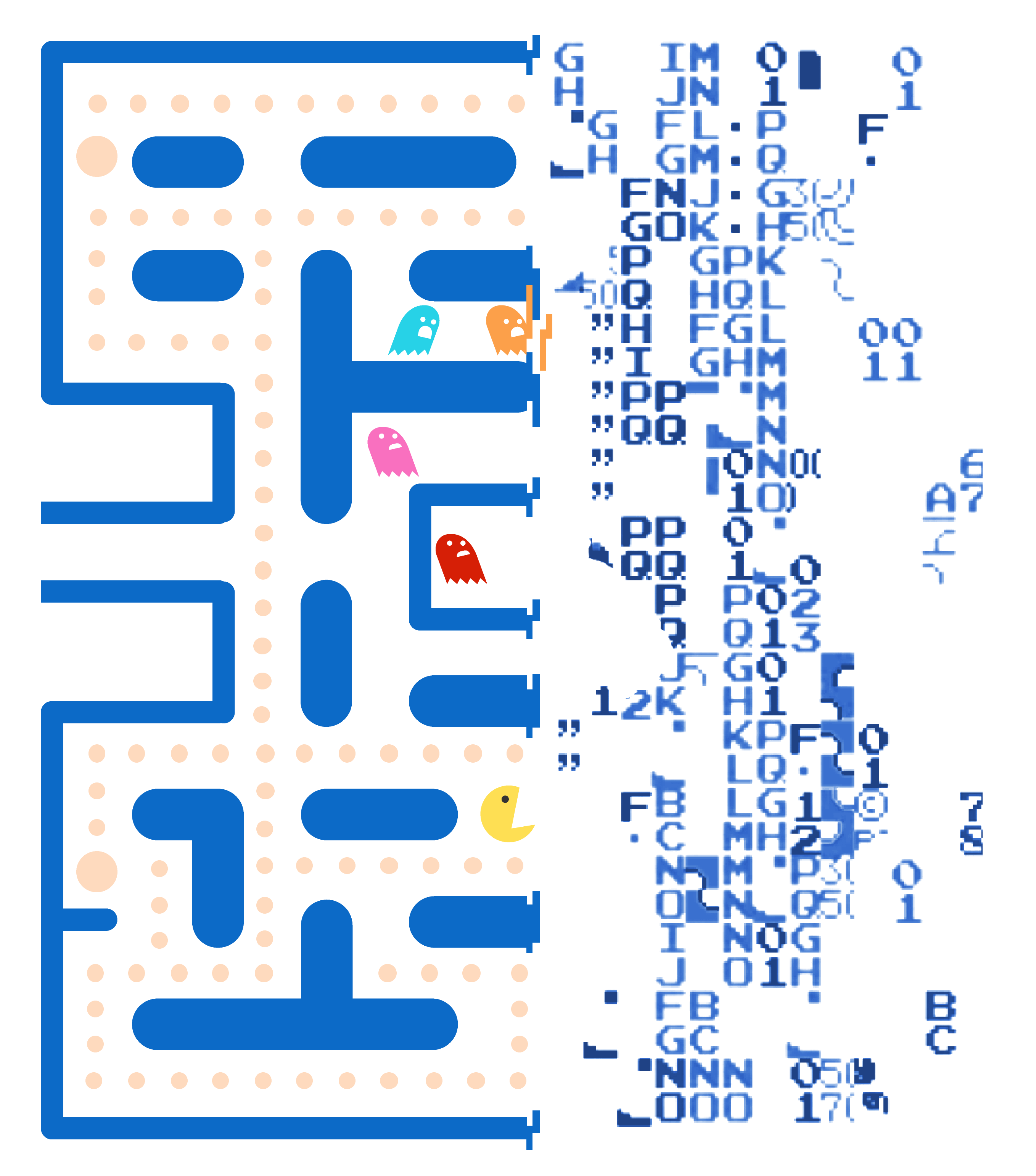### Number Bases

Computers are very good at determining if a switch is on or off. This can be considered akin to a digit being set as 0 or 1. Because of this and the fact that any number can be represented in binary, computers usually use bits (0s and 1s) to store data.

$\large 10110110$

A byte is composed of eight bits; an example byte is shown above. What is the largest number possible (in base 10) of a byte?

# Binary on ComputersSuppose a computer receives a transmission (shown above) and expects to read the first eight bits as a byte, but there is an error such that the first bit is lost. In other words, the computer starts reading at the second rather than the first arrow above. It will still read 8 bits total while attempting to receive the first byte.

What is the difference between the correct number and the number the computer thought it received?

(This kind of issue is why data transmissions always have error checking!)

# Binary on Computers

The ASCII code in common use across nearly every computer consists of 7 bits that encode the letters A to Z (upper and lowercase), the digits 0 to 9, and common punctuation like ; and #. Adding an $8^\text{th}$ bit (making a full byte) extends the ASCII table to some non-English letters and miscellaneous symbols.

In base 10, uppercase A is 65, B is 66, C is 67, and so on to Z at 90.

Lowercase a is 97, b is 98, c is 99, and so on up to z at 122.

It may seem odd that there is a gap between the last uppercase letter and the first lowercase letter, but the gap is there due to the binary system. How many bits (at most) must be swapped from 0 to 1 to turn an ASCII letter from uppercase to lowercase?

# Binary on Computers

Suppose you add 1 to a binary number of length $L$ composed entirely of 1s. What is the result? $($For example, 111 is composed entirely of 1 and has $L = 3 .)$

# Binary on Computers

You have the binary number in computer memory

$11111110$

stored as a byte.

Suppose the computer only retains the eight bit-positions shown above, so if a ninth bit is required to the left of the existing bits, that bit is simply lost. What will the computer think the result of adding two is (given in base 10)?

# Binary on Computers

The issue mentioned in the last question is the source for one of the most infamous video game bugs of all time.In Pac-Man, the "wave" the game is on is stored in a byte. If a player reaches level 255 (not originally anticipated by the developers!) and moves on to level 256, the game gets very confused by incrementing to level 0. Only part of the board gets displayed, and since Pac-Man can't eat the number of dots required to reach the next level, level 256/0 is the "kill screen" for the game.Overflow bugs can be much worse than this! Adding bits to memory locations where they shouldn't be is a common method of hacking past computer security. In other words, binary and related bases like hexadecimal (base 16) have real-world consequences. Start this course now and become a master of binary and beyond!

×